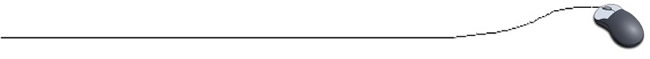Quick SortThe quicksort is considered to be very efficient,  with its "divide and conquer" algorithm.  This sort starts by dividing the original array into two sections (partitions) based upon the value of the first element in the array.  Since our example sorts into descending order, the first section will contain all the elements greater than the first element.  The second section will contain elements less than (or equal to) the first element. It is possible for the first element to end up in either section.  Let's examine our same example:
Array at beginning:  84 69 76 86 94 91
= 1st partition
86 94 91 84 69 76
= 2nd partition
94 91 86 84 69 76
94 91 86 84 69 76
94 91 86 84 69 76
Done: 94 91 86 84 76 69

This sort uses recursion - the process of "calling itself".  Recursion will be studied at a later date.

//Quick Sort Methods for Descending Order
// (2 Methods)
public static void QuickSort(int [ ] num, int top, int bottom)
{

// top = subscript of beginning of array

// bottom = subscript of end of array

int middle;
if (top < bottom)
{
middle = partition(num, top, bottom);
QuickSort(num, top, middle);
// sort first section
QuickSort(num, middle+1, bottom);
// sort second section
}
return;
}

//Method to determine the partitions
// partitions the array and returns the middle subscript
public static int partition(int [ ] array, int top, int bottom)
{
int x = array[top];
int i = top - 1;
int j = bottom + 1;
int temp;
do
{
do
{
j - -;
}while (x >array[j]);

do
{
i++;
} while (x <array[i]);

if (i < j)
{
temp = array[i];
array[i] = array[j];
array[j] = temp;
}
}while (i < j);
return j;
// returns middle subscript
}